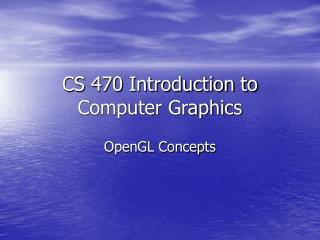DownloadDownload PresentationCS 470 Introduction to Computer Graphics

# CS 470 Introduction to Computer Graphics

Télécharger la présentation## CS 470 Introduction to Computer Graphics

- - - - - - - - - - - - - - - - - - - - - - - - - - - E N D - - - - - - - - - - - - - - - - - - - - - - - - - - -
##### Presentation Transcript

1. CS 470 Introduction to Computer Graphics OpenGL Concepts

2. Opengl – several related components • OpenGL • glu • glut (freeglut, glow, glutmaster(c++)…) • Others • glx • wgl • apl • pgl • GLUI

3. gl • 200 functions • Primitives • Geometric • Discrete (bitmaps) • Attribute functions • Viewing functions

4. glu – Opengl Utility Library • GLU is the OpenGL Utility Library. This is a set of functions to create texture bitmaps from a base image, map coordinates between screen and object space, … • about 17 functions • about 4 drawing primitives

5. glut – Opengl Utility Toolkit • …a window system independent toolkit for writing OpenGL programs • Generic windows API – • same program/calls work across different gui/windows environments • Provides user interaction functions • mouse, keyboard, menus

6. Sample Drawing Primitives • gl • Pixels • Bitmaps • Vertice • glu • Cylinder • Disk • Sphere

7. Sample Drawing Primitives • glut • wire cube / solid cube • wire sphere / solid sphere • wire octahedron / solid octahedron • wire torus / solid torus • wire teapot / solid teapot

8. OpenGL • Written in C • No overloading of functions • No object oriented programming • but be used from C++

9. OpenGL Function syntax • glxxxx*() – all opengl functions begin with “gl” • gluxxx*() – all glu functions begin with “glu” • glutxxx*() – all glut functions begin with “glut”

10. Other libraries syntax • glx – opengl library for X windows • wgl – opengl library for MS Windows • agl – opengl library for Apple Macintosh OS • pgl – opengl library for OS/2 Warp glxCreateWindow() Not same across libraries

11. OpenGL • general function syntax • glVertex{2|3|4}{s|i|f|d}(TYPE arg1,…); • glVertex{2|3|4}{s|i|f|d}v(TYPE *arg); • glVertex*() manual notation

12. OpenGL • Data types • standard data types – int, float, double, char • OpenGL types • GLbyte – signed char • GLushort – unsigned short • GLint – int • GLuint – unsigned int • GLfloat – float • GLdouble – double • GLchar – char

13. OpenGL • Other Data Types – • GLclampf – float from 0.0 to 1.0 • GLsizei – largest nonnegative integer on system • other enumerated GL types • Also some GLU types

14. OpenGL • OpenGL constants • Predefined constants for setting or testing values • GL_RGB • GL_LINE • GL_POLYGON • GLUT_RGB • GLUT_SINGLE • GLUT_KEY_F1 • GLUT_LEFT_BUTTON • Hundreds of them

15. OpenGL • OpenGL rendering pipeline • simplified pipeline model Primitive Generation Transform-ation Clipping Projection Rasterization Display

16. OpenGL as a state machine • OpenGL can be viewed as a state-machine • The action of a function depends on the machines current state • Hundreds of properties (state variables) define the state of OpenGL • A state is in effect until it is changed • glColor3f(1.0,0.0,0.0) • State variables not like object properties

17. OpenGL Buffers • Frame Buffers • Single • Double